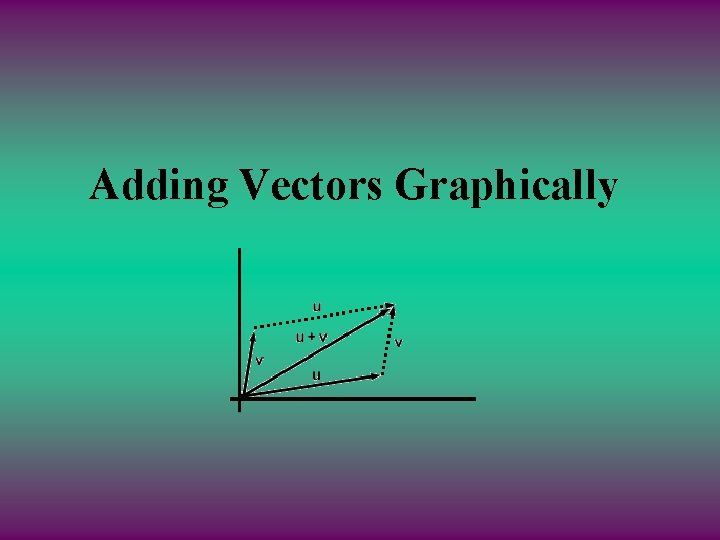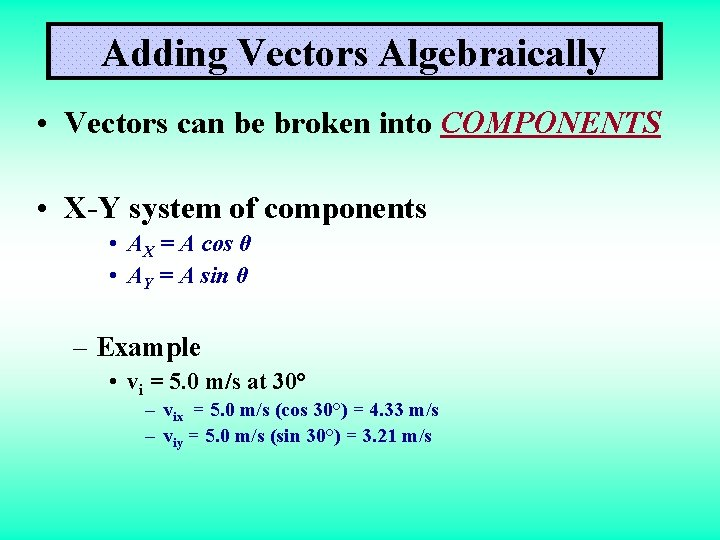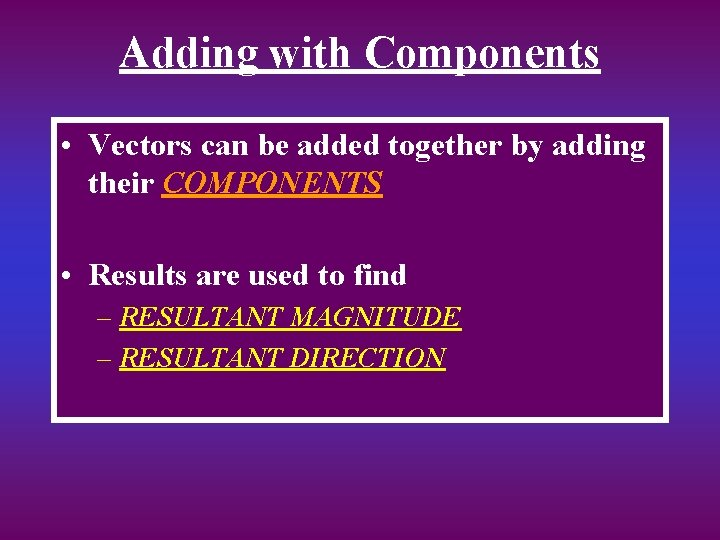# Pointing the Way Vectors Representing Vectors Vectors on

• Slides: 8Pointing the Way Vectors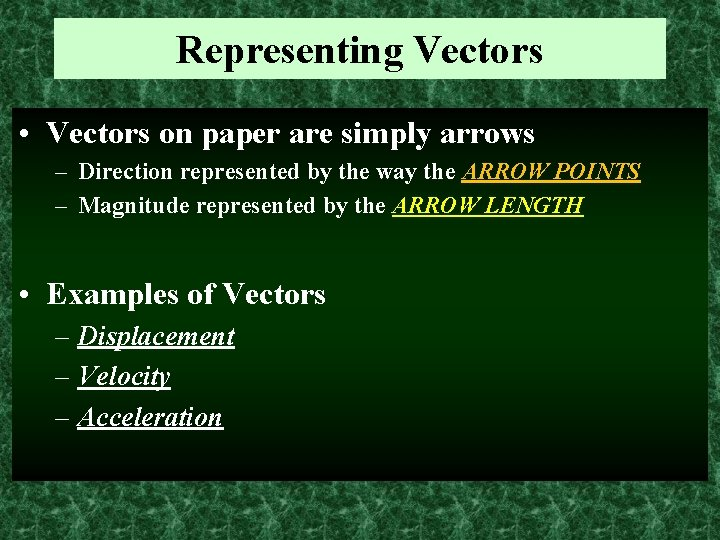Representing Vectors • Vectors on paper are simply arrows – Direction represented by the way the ARROW POINTS – Magnitude represented by the ARROW LENGTH • Examples of Vectors – Displacement – Velocity – Acceleration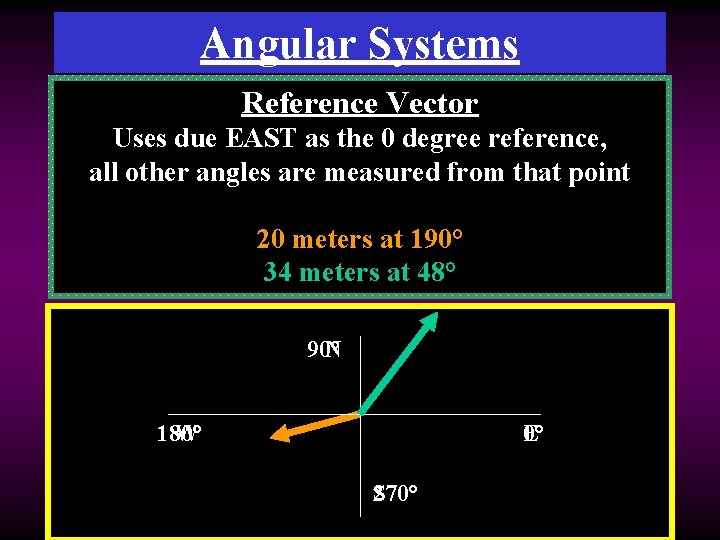Angular Systems Compass Reference Point Vector System Usesangles due EAST measured as thefrom 0 degree various reference, compass all other points angles to reference are measured vectorfrom direction that point 20 meters at 10° at south 190°of west 34 meters at 42° east at 48° of north 90° N 180° W E 0° S 270°Changing Systems • What is the reference vector angle for a vector that points 50 degrees east of south? 270° + 50° = 320° 50° • What is the reference vector angle for a vector that points 20 degrees north of east? 20°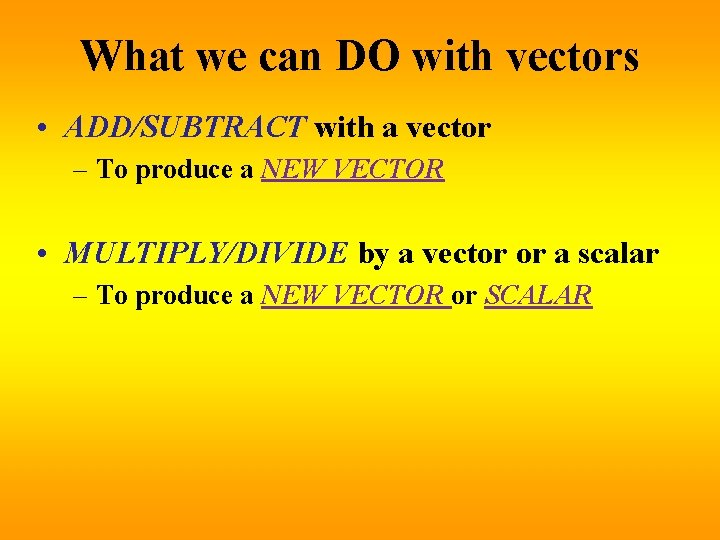What we can DO with vectors • ADD/SUBTRACT with a vector – To produce a NEW VECTOR • MULTIPLY/DIVIDE by a vector or a scalar – To produce a NEW VECTOR or SCALAR